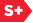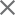### Proceedings Paper

Lattice quantization in the wavelet domain
Author(s): William C. Powell; Stephen G. Wilson
Format Member Price Non-Member Price
PDF \$17.00 \$21.00

Paper Abstract

Some of the most remarkable image compression results published recently are the Barlaud et al, where an entropy estimate is used as the measure of coded bit rate. The rates they quoted are not achievable in a real image compression system because the number of codewords in the lattice vector quantizer codebook used by Barlaud et al. can be orders of magnitude greater than the number of quantizer source vectors output from the wavelet transform of a single image. The root of this problem is that lattice vector quantizers are best suited for use with uniform source probability distributions. In this paper we propose a novel quantizer that compands the codebook lattice in a piecewise fashion, is based on the independent Laplacian source model of wavelet transform coefficients, and achieves excellent rate-distortion results for quantization of wavelet transform coefficients. Simulations compare the use of this quantizer on wavelet transform coefficients with the quantizer originally proposed by Barlaud et al.

Paper Details

Date Published: 1 November 1993
PDF: 12 pages
Proc. SPIE 2034, Mathematical Imaging: Wavelet Applications in Signal and Image Processing, (1 November 1993); doi: 10.1117/12.162066
Show Author Affiliations
William C. Powell, Microsoft Corp. (United States)
Stephen G. Wilson, Univ. of Virginia (United States)

Published in SPIE Proceedings Vol. 2034:
Mathematical Imaging: Wavelet Applications in Signal and Image Processing
Andrew F. Laine, Editor(s)